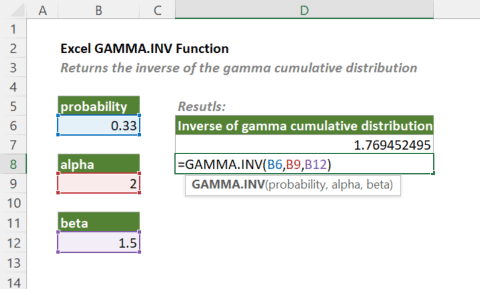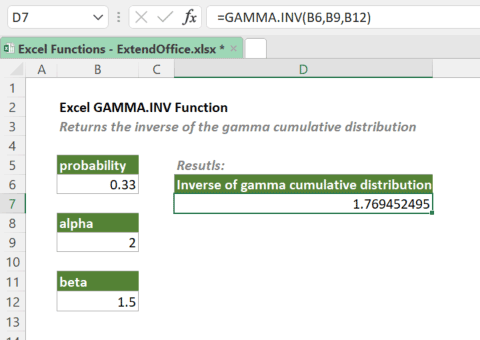## Excel GAMMA.INV Function

The GAMMA.INV function returns the inverse of the gamma cumulative distribution. The function is frequently used to provide probabilities for values that may have a skewed distribution, such as queuing analysis.

Note: The GAMMA.INV function was introduced in Excel 2010. So, it is not available in earlier versions of Excel. For users of earlier Excel versions, please use the GAMMAINV function.#### Syntax

=GAMMA.INV(probability, alpha, beta)

#### Arguments

• probability (required): The probability for which to evaluate the inverse gamma cumulative distribution. Note: probability must satisfy the equation: 0 ≤ probability < 1.
• alpha (required): A parameter of the distribution. Note: alpha must be positive.
• beta (required): A parameter of the distribution. If beta = 1, then the standard gamma distribution is calculated. Note: beta must be positive.

#### Return Value

The GAMMA.INV function returns a numeric value.

#### Function Notes

• GAMMA.INV returns the #NUM! error if any of the below conditions is met:
• probability < 0 or probability ≥ 1;
• alpha ≤ 0;
• beta ≤ 0.
• GAMMA.INV returns the #VALUE! error if probability, alpha, or beta is not a numeric value.

#### Example

To calculate the value of the inverse gamma cumulative distribution for the given probability, alpha and beta values, please copy or enter the formula below in the result cell and then press Enter to get the result.

=GAMMA.INV(B6,B9,B12)Also, you can type the actual probability, alpha and beta values in the formula as shown below.

=GAMMA.INV(4,2,1.5)

#### GAMMA.INV Vs. GAMMA.DIST

The Excel GAMMA.INV function is the inverse of the GAMMA.DIST function (when used to calculate the cumulative distribution function, i.e., the cumulative argument is set to TURE). For a supplied value for probability, GAMMA.INV(probability, alpha, beta) returns the value of x such that probability = GAMMA.DIST(x, alpha, beta, TRUE).

#### Related functions

Excel GAMMAINV Function

The GAMMAINV function returns the inverse of the gamma cumulative distribution. The function is frequently used to provide probabilities for values that may have a skewed distribution, such as queuing analysis.

Excel GAMMA Function

The GAMMA function returns the value of the gamma function for a specified number.

The GAMMADIST function returns the gamma distribution. The function is frequently used to provide probabilities for values that may have a skewed distribution, such as queuing analysis.

### The Best Office Productivity Tools

#### Kutools for Excel - Helps You To Stand Out From Crowd

 Popular Features: Find, Highlight or Identify Duplicates  |  Delete Blank Rows  |  Combine Columns or Cells without Losing Data  |  Round without Formula ... Super VLookup: Multiple Criteria  |  Multiple Value  |  Across Multi-Sheets  |  Fuzzy Lookup... Adv. Drop-down List: Easy Drop Down List  |  Dependent Drop Down List  |  Multi-select Drop Down List... Column Manager: Add a Specific Number of Columns  |  Move Columns  |  Toggle Visibility Status of Hidden Columns  |  Compare Columns to Select Same & Different Cells ... Featured Features: Grid Focus  |  Design View  |  Big Formula Bar  |  Workbook & Sheet Manager | Resource Library (Auto Text)  |  Date Picker  |  Combine Worksheets  |  Encrypt/Decrypt Cells  |  Send Emails by List  |  Super Filter  |  Special Filter (filter bold/italic/strikethrough...) ... Top 15 Toolsets:  12 Text Tools (Add Text, Remove Characters ...)  |  50+ Chart Types (Gantt Chart ...)  |  40+ Practical Formulas (Calculate age based on birthday ...)  |  19 Insertion Tools (Insert QR Code, Insert Picture from Path ...)  |  12 Conversion Tools (Numbers to Words, Currency Conversion ...)  |  7 Merge & Split Tools (Advanced Combine Rows, Split Excel Cells ...)  |  ... and more

Kutools for Excel Boasts Over 300 Features, Ensuring That What You Need is Just A Click Away...#### Office Tab - Enable Tabbed Reading and Editing in Microsoft Office (include Excel)

• One second to switch between dozens of open documents!
• Reduce hundreds of mouse clicks for you every day, say goodbye to mouse hand.
• Increases your productivity by 50% when viewing and editing multiple documents.
• Brings Efficient Tabs to Office (include Excel), Just Like Chrome, Edge and Firefox.